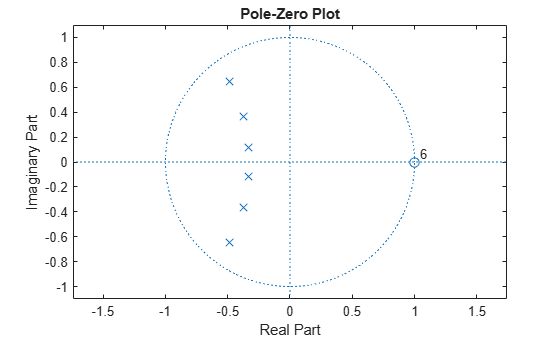# isstable

Determine whether filter is stable

## Syntax

``flag = isstable(b,a)``
``flag = isstable(sos)``
``flag = isstable(d)``

## Description

example

````flag = isstable(b,a)` returns a logical output equal to `1` if the filter specified by numerator coefficients `b` and denominator coefficients `a` is stable.```

example

````flag = isstable(sos)` returns `1` if the filter specified by second order sections matrix `sos` is stable.```
````flag = isstable(d)` returns `1` if the digital filter `d` is stable. Use `designfilt` to generate `d` based on frequency-response specifications.```

## Examples

collapse all

Design a sixth-order Butterworth highpass IIR filter using second order sections. Specify a normalized 3-dB frequency of 0.7. Determine if the filter is stable.

```[z,p,k] = butter(6,0.7,"high"); SOS = zp2sos(z,p,k); flag = isstable(SOS) ```
```flag = logical 1 ```
`zplane(z,p)`Redesign the filter using `designfilt` and check it for stability.

```d = designfilt("highpassiir",DesignMethod="butter",FilterOrder=6, ... HalfPowerFrequency=0.7); dflg = isstable(d)```
```dflg = logical 1 ```
`zplane(d)`Create a filter and determine its stability at double and single precision.

```b = [1 -0.5]; a = [1 -0.999999999]; act_flag1 = isstable(b,a)```
```act_flag1 = logical 1 ```
`act_flag2 = isstable(single(b),single(a))`
```act_flag2 = logical 0 ```

## Input Arguments

collapse all

Filter coefficients, specified as vectors.

Data Types: `single` | `double`

Second order sections, specified as a k-by-6 matrix where the number of sections k must be greater than or equal to 2. Each row of `sos` corresponds to the coefficients of a second order (biquad) filter. The ith row of the matrix corresponds to ```[bi(1) bi(2) bi(3) ai(1) ai(2) ai(3)]```.

Data Types: `double`

Digital filter, specified as a `digitalFilter` object.

## Output Arguments

collapse all

Logical output, returned as `1` or `0`. If the poles lie on or outside the circle, the function returns `0`. If the poles are inside the circle, the function returns `1`.

Data Types: `logical`

## Version History

Introduced in R2013a